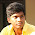## Saturday, 4 August 2012

### TRIANGULAR WAVE GENERATOR USING OP-AMP

Here am going to explain generation of triangular wave using 741 op-amp. Triangular waves can also be generated by integrating the output of symmetrical square wave generated by the astable multivibrator. The triangular waves are used for studies.
This circuit uses two operational amplifiers. Op-amp A1 fuctions as a comparator and op-amp A2 as an integrator. Comparator compares the voltage at point P continuisly with respect to the voltage at the inverting input, which is grounded potential. When the voltage at P goes slightly below zero , the output of A1 will switch to negative satuaration.

When the output of A1 is at positive saturation +Vsat, this voltage is the input of the integrator, the output of A2 will be a negative going ramp. Thus one end of the voltage devider R1-R2 is at +Vsat and other at the negative going ramp. At time t=t1 ; when the -ve going ramp atains value of -Vramp the effective voltage at point P becomes slightly less than 0 V. This produce output of A1 from positive saturation to negative saturation level -Vsat.

During the time when the output of A1 is at -Vsat , the output of A2 increases in positive direction. At the time t=t2 the voltage at point P becomes just above 0 V, there by switching the output of A1 from -Vsat to +Vast . The cycle repeat and generate triangular waveform. The frequency of triangular wave isf=R1/4 R2 R3 C

Components for wiring this circuit

Op-amp ic 741C - 2
R1 - 1K
R2 - 180 Ω
R3 - 12K
C - 0.1μF

1.This is one of the simplest and cleanest triangle generator circuits I've seen. I wonder, could you post one using a single supply? Also, a way to control the DC offset?

1.thankx

if u want dis generator to be in a single supply...., den rearrange the circuit as....give one supply voltage common to both opamps....

2.2.frequency control through the circuit parameters of resistances and capacitances...could you post that...

3.why you connected R2 to output of 2nd opamp.Is that necessary???

1.yup. it is called shunt resistor

4.How about just using a voltage divider (equal value resistors) and putting the Vcc/2 where the grounds are on both op amps now. That would create a DC offset in the output, but would not require two supply voltages. And the offset could be removed by just using a coupling capacitor in the output.

1.ya thats right too